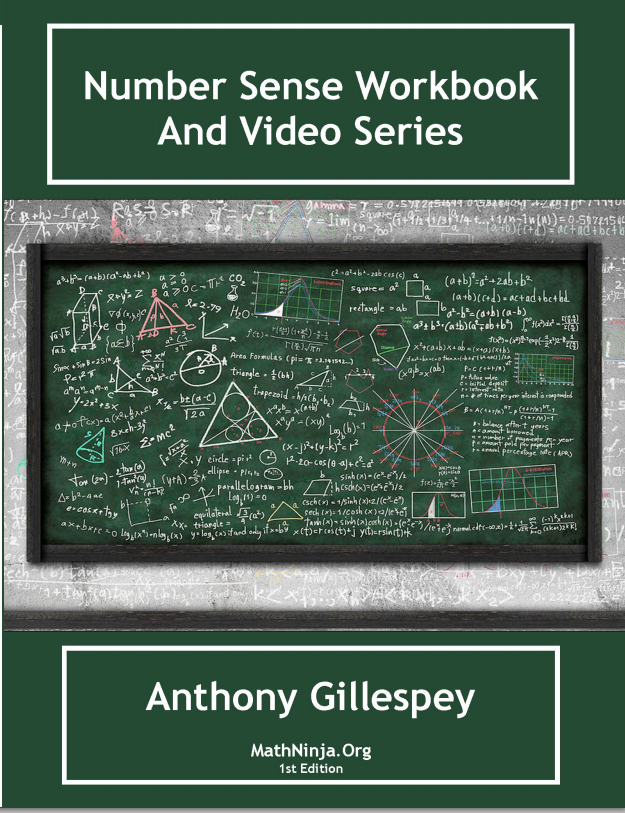# Number Sense Workbook and Video Lessons Volume 1

## eo, do the extra problems on each page of the workbook.  There is a key In the back of the booklet for each section.  I just added a new page for Errata in the book at http://mathninja.org/number-sense-workbook-errata/## A sample of the booklet can be found  here.

Sample Number sense booklet## Pages 5 through 25

Page 5 – Add to 10 Ones Digit Page 6- Add to 10 Tens Digit Page 7 – Multiplying by 25 Page 8 – Multiplying by 50 Page 9 – Multiplying by 75 Page 10 – Multiplying by 125 Page 11 – Multiplying by 11 Page 12 – Multiplying by 111 Page 13 – Multiplying …

## Pages 26 through 46

Pg 26 – Approximating multiplying by 142857 Pg 27 – Multiplying by 3367 Pg 28 – Multiplying by 143 Pg 29 – Add to 1 Mixed Numbers Pg 30 – Difference of Two Squares Pg 31 – Sum of Two Squares Pg 32 – Greatest Common Factor Pg 33 – Least Common Multiple Pg 34 – …

## Pages 47 through 65

Page 47 – Simplifying Radicals Page 48 – % of is % of what number Page 49 – Multiplying powers of pi Page 50 – Powers of i Page 51 – If a= b and b = c then 1a = ? c Page 52 – Sum/Product of the Roots and Discriminate Page 53 – Log …

#### 65 pings

1. ##### Number Sense Booklet is Up!! | MathNinja.org

[…] Number Sense Video Lessons […]